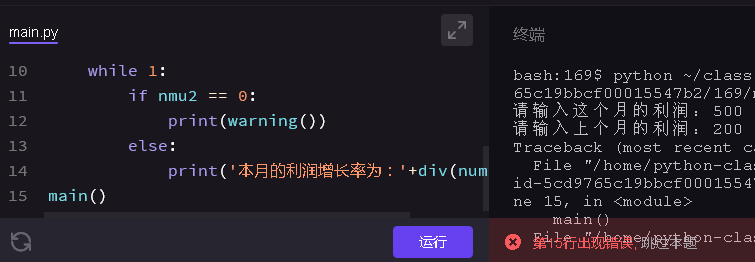python 这段代码我这里为什么说15行出现错误呢
``````def div(num1,num2):
growth = (num1-num2)/num2
percent = str(growth*100) + '%'
return percent
def warning():
print('警告：你确定上个月不亏也不赚吗？')
def main():
num1 = int(input('请输入这个月的利润：'))
num2 = int(input('请输入上个月的利润：'))
while 1:
if nmu2 == 0:
print(warning())
else:
print('本月的利润增长率为：'+div(num1,num2))
main()
``````2个回答

``````  while 1:
if nmu2 == 0:
print(warning())
else:
print('本月的利润增长率为：'+div(num1,num2))
``````5 个月之前 回复5 个月之前 回复

num2不是nmu2``` def test(got, expected): if got == expected: prefix = '正确!' else: prefix = '错误!' print('%s 你的结果: %s 应该返回的结果: %s' % (prefix, repr(got), repr(expected))) def match_ends(words): for item in match_ends: if len(item)>2 and item==item[-1]: number = number+1 else : continue return test(match_ends(['aba', 'xyz', 'aa', 'x', 'bbb']), 3) test(match_ends(['', 'x', 'xy', 'xyx', 'xx']), 2) test(match_ends(['aaa', 'be', 'abc', 'hello']), 1) ``` 其中错误提示是： ``` TypeError Traceback (most recent call last) <ipython-input-59-28f9cc682b29> in <module> 14 return 15 ---> 16 test(match_ends(['aba', 'xyz', 'aa', 'x', 'bbb']), 3) 17 test(match_ends(['', 'x', 'xy', 'xyx', 'xx']), 2) 18 test(match_ends(['aaa', 'be', 'abc', 'hello']), 1) <ipython-input-59-28f9cc682b29> in match_ends(words) 7 8 def match_ends(words): ----> 9 for item in match_ends: 10 if len(item)>2 and item==item[-1]: 11 number = number+1 TypeError: 'function' object is not iterable ``` 该如何将这个函数更改为可迭代的呢？

python的错误异常捕获失效问题

**问题描述：想用python程序实现一个登陆验证用户名密码的效果，但是我修改前的python代码与我想象中的不太一样，修改后的python代码与我预想的执行结果(红色代码最后输出)一致，但是却不知其所以然，求大神解答** 运行环境：Windows，pycharm ## **修改前的python代码如下：** ``` user_n = "user" user_p = "123" bit = False for i in range(3): user_name = str(input("请输入账户名：")) user_password = str(input("请输入密码：")) if user_name == user_n and user_password == user_p: bit = True print("登录成功，欢迎回来") exit() else: print("用户名密码错误，请重新输入") if not bit: exit("尝试次数超过限制") ``` ## 我预想的执行结果 ![图片说明](https://img-ask.csdn.net/upload/201901/26/1548480347_659870.jpg) ## 而我实际的执行结果 ![图片说明](https://img-ask.csdn.net/upload/201901/26/1548480506_562785.jpg) ## **修改后的python代码与我的预想执行结果一致，代码如下：** ``` user_n = "user" user_p = "123" for i in range(3): user_name = str(input("请输入账户名：")) user_password = str(input("请输入密码：")) if user_name == user_n and user_password == user_p: print("登录成功，欢迎回来") exit() else: print("用户名密码错误，请重新输入") else: exit("尝试次数超过限制") ``` 

Python返回函数不理解之处

#include<stdio.h> int main() { void convert(int n); int n,i; char a; scanf("%d",&n); convert(n); printf("%s\n",a[i]); return 0; } void convert(int n) {int s; char c; if ((s=n/10)>=1) {c=n%10+'0'; n=(int)s; convert(n); } }

from matplotlib.finance import quotes historical_yahoo from datetime import date import pandas as pd today=date.today() start=(today.year-1,today.month,today.day) quotes=quotes_historical_yahoo('AXP',start,today) df=pd.DataFrame(quotes) print df

python一个关于while循环的报错问题

girls = ['gujin','yangmi','zhengshuang'] my_girls = [] a = len(girls) a = int(a) while a >= 0: a=a-1 girl = girls.pop() my_girls.append(girl) continue for my_girl in my_girls: print(my_girl) 报错： Traceback (most recent call last): File "girls4.py", line 6, in <module> girl = girls.pop() IndexError: pop from empty list 这段执行不报错： girls = ['gujin','yangmi','zhengshuang'] my_girls = [] while girls: girl = girls.pop() my_girls.append(girl) for my_girl in my_girls: print(my_girl)

Python脚本运行问题，在pycharm中运行会出错

python字典配合占位符出错

a={'name':'xiaoming','age':'18'} b=a['name'] print (b) c=a['age'] print ('%%s的年龄是%s岁'%b%c) 这段代码，在打印时在第一个占位符处多加了个%,打印出来的结果成了18的年龄是xiaoming岁。颠倒了，打印过b和c的值。确实也是我想要的，可组合后，结果却变 了，不多加%，报not enough arguments for format string错误。我知道把b和c，调换下位置就是我想要的结果。但是我想弄明白。多加一个%为什么就颠倒了。不加百分号又报错的原因是什么，怎么解决。 a={'name':'xiaoming','age':18} print ('%s的年龄是%s岁'%a['name]%a['age']) 试过这样写，提示语法错误。为什么元素是列表时配合着使用下标又可以。求大神赐教

“亚马逊丛林里的蝴蝶扇动几下翅膀就可能引起两周后美国德州的一次飓风……” 这句人人皆知的话最初用来描述非线性系统中微小参数的变化所引起的系统极大变化。 而在更长的时间尺度内，我们所生活的这个世界就是这样一个异常复杂的非线性系统…… 水泥、穹顶、透视——关于时间与技艺的蝴蝶效应 公元前3000年，古埃及人将尼罗河中挖出的泥浆与纳特龙盐湖中的矿物盐混合，再掺入煅烧石灰石制成的石灰，由此得来了人...

loonggg读完需要3分钟速读仅需 1 分钟大家好，我是你们的校长。我之前讲过，这年头，只要肯动脑，肯行动，程序员凭借自己的技术，赚钱的方式还是有很多种的。仅仅靠在公司出卖自己的劳动时...

MySQL数据库面试题（2020最新版）

HashMap底层实现原理，红黑树，B+树，B树的结构原理 Spring的AOP和IOC是什么？它们常见的使用场景有哪些？Spring事务，事务的属性，传播行为，数据库隔离级别 Spring和SpringMVC，MyBatis以及SpringBoot的注解分别有哪些？SpringMVC的工作原理，SpringBoot框架的优点，MyBatis框架的优点 SpringCould组件有哪些，他们...

《经典算法案例》01-08：如何使用质数设计扫雷（Minesweeper）游戏

《Oracle Java SE编程自学与面试指南》最佳学习路线图（2020最新版）